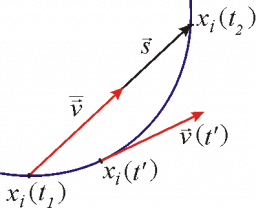A stretch

A stretch of high way that is 12 1/4 kilometers long has speed limit signs every 7/8 of a kilometer. How many speed limit signs are on this stretch highway?

n =  14/1 = 14

Step-by-step explanation:Did you find an error or inaccuracy? Feel free to write us. Thank you!Tips to related online calculators
Need help to calculate sum, simplify or multiply fractions? Try our fraction calculator.
Do you want to convert length units?
Do you want to round the number?